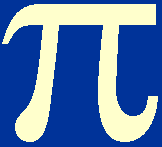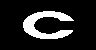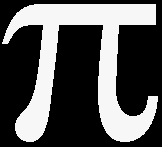There are six fundamental properties of stars which can be readily determined by observation. These are:

1. mass (Ms)
2. luminosity (Ls)
defined as the total energy radiated per second, i.e. the power, from a star.
4. effective temperature (Te)
defined as the temperature of the black body of the same size as the star that would emit the same total power. The effective temperature is related to the luminosity and the radius of the star by

Ls = 4rs2Te4

whereis the Stefan-Boltzmann constant.
5. age
6. chemical composition (X, Y, Z)
where X, Y, Z are the fractional proportions, by mass, of hydrogen, helium and metals.

Table 1 lists how each of these properties are determined and gives the range of their values in terms of the properties of the Sun (denoted by the subscript- click onfor a list of solar values).

 Table 1: Six fundamental properties of stars, how they are determined from observation and the range in their values.

 property determined from range of values mass (Ms) binary stars  or g (spectrum) and Rs 10-1 M<  Ms < 50 Mluminosity (Ls) apparent magnitude and distance  or spectrum (luminosity class) 10-4 L<  Ls < 106 Lradius (rs) Ls, Te [Ls = 4rs2Te4] or interferometry (angular diameter) and distance or eclipsing binary stars 10-2 r<  rs < 103 reffective temperature (Te) continuous spectrum  or spectral type 2 × 103 K <  Te < 105 K age star clusters and theory 0 - 1010 y chemical composition (X, Y, Z) line spectrum X=0.747  Y=0.236  Z=0.017

Note that the very high luminosities of exploding supernovae and the very low luminosities of neutron stars have been omitted from the above limits, as have the properties of brown dwarfs. For detailed definitions of the above properties and the techniques of measurement, see chapter 2 of Tayler.